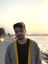Search
•Inan Evin

# #12 Lina Engine: Math Library - Part 3 Matrix4

There is no doubt that we will need a solid Matrix4 class that is able to perform matrix multiplication, as well as other operations to produce resulting matrices. We will use Shaders to represent active transformations of objects in 3D game spaces. We will pass in vertices and indices to OpenGL to draw, and we are going to bind particular shaders to it in order to define how to draw those vertices. I will talk about mesh rendering and shaders on the future posts, but for now, I just wanted to point out the top-priority matrix calculations for our engine.

Initially, we will be representing our objects in shaders as 4 by 4 matrices. We will have only one transformation matrix to manipulate where we draw our vertices in shader. However, this single transformation matrix will be the result of multiple matrix calculations, the ingredients for this calculation is most basically; scale matrix, position matrix and rotation matrix. The 4x4 matrix representation has a very interesting ideology to grasp, and I will try my best to tell you about it in the means of 3D space rendering. So, let's only talk about scaling an object for now. Assume that we have x-y-z components for the scale on the object. Let's take a look at the scale matrix for this scenario:

"m" is our 4x4 matrix here. The first for, represented with the 0 index element, m[y] is the X component of our scale. The whole row. Every single column in that row, represents the "power" of the corresponding element, on the mentioned row. So basically, m is the power of x component, on the scale on X axis. m is the power of Y component, on the scale on X axis. m is the power of X component on the scale of Z axis and so on. So as you can see we use 4x4 matrix to represent various impacts of each component, on each axis of our performing transformation.

Just like the scale function above, I have also implemented position, rotation and multiplication calculations for out Matrix4 library. You can take a look at them if you please below, and see you on the next post!